Download PresentationHomogeni z ation and porous media

# Homogeni z ation and porous media - PowerPoint PPT Presentation

Homogeni z ation and porous media. by Ulrich Hornung Chapter 1: I ntroduction. Heike Gramberg , CASA Seminar Wednesday 23 February 2005. Overview. Diffusion in periodic media Special case: layered media Diffusion in media with obstacles Stokes problem: derivation of Darcy’s law.I am the owner, or an agent authorized to act on behalf of the owner, of the copyrighted work described.
Download Presentation## Homogeni z ation and porous media

An Image/Link below is provided (as is) to download presentation

Download Policy: Content on the Website is provided to you AS IS for your information and personal use and may not be sold / licensed / shared on other websites without getting consent from its author.While downloading, if for some reason you are not able to download a presentation, the publisher may have deleted the file from their server.

- - - - - - - - - - - - - - - - - - - - - - - - - - E N D - - - - - - - - - - - - - - - - - - - - - - - - - -
Presentation Transcript### Homogenization and porous media

by Ulrich Hornung

Chapter 1: Introduction

Heike Gramberg, CASA Seminar Wednesday 23 February 2005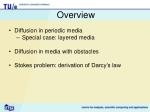Overview
• Diffusion in periodic media
• Special case: layered media
• Diffusion in media with obstacles
• Stokes problem: derivation of Darcy’s law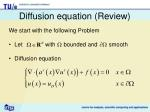Diffusion equation (Review)
• We start with the following Problem
• Let with bounded and smooth
• Diffusion equation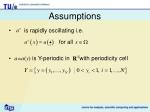Assumptions
• is rapidly oscillating i.e.
• a=a(y) is Y-periodic in with periodicity cell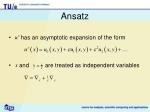Ansatz
• has an asymptotic expansion of the form
• and are treated as independent variables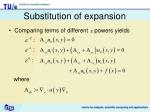Substitution of expansion
• Comparing terms of different powers yields
• where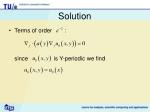Solution
• Terms of order :
• since is Y-periodic we find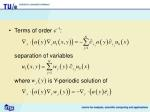Terms of order :

separation of variables

where is Y-periodic solution of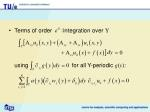Terms of order integration over Y

• using for all Y-periodic g(y):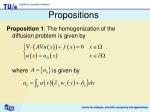Propositions

Proposition 1: The homogenizationof the

diffusion problem is given by

where is given byProposition 2:
• The tensor A is symmetric
• If a satisfies a(y)>a>0 for all y then

A is positive definiteRemarks
• are uniquely defined up to a constant
• are uniquely defined
• Problem can be generalized by considering
• Eigenvalues l of A satisfy Voigt-Reiss inequality:

whereExample: Layered Media
• Assumption:
• Then

and is Y-periodic solution ofProposition 3:
• If , then
• The coefficients are given byRemarks
• Effective Diffusivity in direction parallel to layers is given by arithmeticmean of a(y)
• Effective Diffusivity in direction normal to layers is given by geometricmean of a(y)
• Extreme example of Voigt-Reiss inequalitye

Media with obstacles
• Medium has periodic arrangement of obstaclesFormal description of geometry
• Standard periodicity cell
• Geometric structure within
• Assumption:Diffusion problem
• Diffusion only in
• Assumptions: andSubstitution of expansion
• Comparing terms of different powers yieldsLemmas
• Lemma 1: for and
• Lemma 2 (Divergence Theorem):

for Y-periodicSolution
• Terms of order : for
• using Lemmas 1 and 2 we find
• thereforeTerms of order : for
• with boundary condition forseparation of variables

where is Y-periodic solution ofTerms of order

• using Lemma 2 and boundary conditions:
• hence is solution ofProposition

Proposition 4: The homogenizationof the

diffusion problem on geometry with obstacles is given by

where is given byRemarks
• Due to the homogeneous Neumann conditions on integrals over boundary disappear
• Weak formulation of the cell problem

where is characteristic function ofStokes problem
• For media with obstacles
• AssumptionsSolution
• Comparing coefficients of the sameorder
• Stokes equation:
• Conservation of mass:
• Boundary conditions:• Separation of variables for both

where are solution ofDarcy’s law
• Averaging velocity over

where is given byConservation of mass
• Term of order in conservation of mass
• Integration over yieldsProposition

Proposition 5: The homogenization of the Stokes problem is given by

Proposition 6: The tensorKis symmetric and positive definiteConclusions
• We have looked at homogenization of the Diffusion problem and the Stokes problem on media with obstacles
• Solutions of the homogenized problems can be expressed in terms of solutions of cell problems
• The homogenization of the Stokes problem leads to the derivation of Darcy’s law